GeeksforGeeks App
Open AppBrowser
Continue

# Division Algorithm Problems and Solutions

Polynomials are made up of algebraic expressions with different degrees. Degree-one polynomials are called linear polynomials, degree-two are called quadratic and degree-three are called cubic polynomials. Zeros of these polynomials are the points where these polynomials become zero. Sometimes it happens that we have some zeros of the polynomials, we need to find the other zeros. For example, let’s assume a polynomial p(x) = x3 – 3x2 – x + 3, and we know that one of the zeros is 1. Then x – 1 must be a factor of this polynomial. The goal is to find the other two zeros. In such cases, the division algorithm helps us.

## Division Algorithm for Polynomials

Let’s have two polynomials p(x) and g(x), and g(x) ≠ 0. Now we can find two polynomials q(x) and r(x) such that,

p(x) = q(x) x g(x) + r(x),

Here, either r(x) = 0 or degree of r(x) < degree of g(x). This is called a polynomial division algorithm for polynomials.

Dividend = Quotient x Divisor + Remainder

### Steps for long division:

Now let’s see with an example, how to divide two polynomials, Let’s say we have p(x) = 2x2 + 4x + 1 and g(x) = x + 1.

• We will stop this process when the remainder becomes zero, or its degree becomes less than the divisor.
• Quotients’ first term is obtained by dividing the highest order term of dividend with the highest degree term of the divisor.
• For the second term, divide the highest degree term of the new dividend obtained as remainder by the highest degree term of the divisor.
• Continue the steps until the condition mentioned in step 1 is met.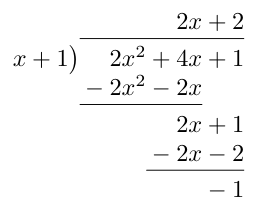Notice that in this example, q(x) = 2x + 2 and r(x) = -1.

## Sample Problems

Question 1: Given the polynomial p(x) = x2 + x +5 and g(x) = x +2. Find the value of q(x) and r(x).

Solution:

Using the steps mentioned above. On dividing p(x) with g(x) we get,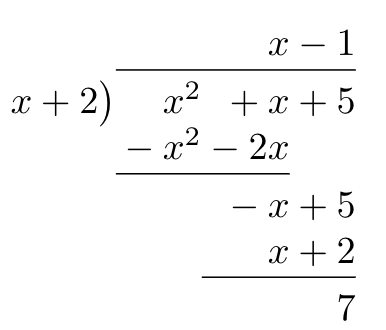q(x) = x – 1 and r(x) = 7.

Question 2: Given the polynomial p(x) = x3 + x + 6x2 + 4 and g(x) = x2 + 1. Find the value of q(x) and r(x).

Solution:

Using the steps mentioned above. On dividing p(x) with g(x) we get,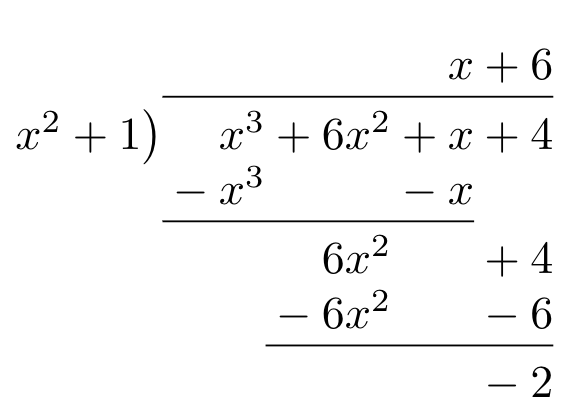So, here q(x) = x + 6, and r(x) = -2.

Question 3: Given the polynomial p(x) = x4 + x + 6x2 + 4 and g(x) = x2 + 1. Find the value of q(x) and r(x).

Solution:

Using the steps mentioned above. On dividing p(x) with g(x) we get,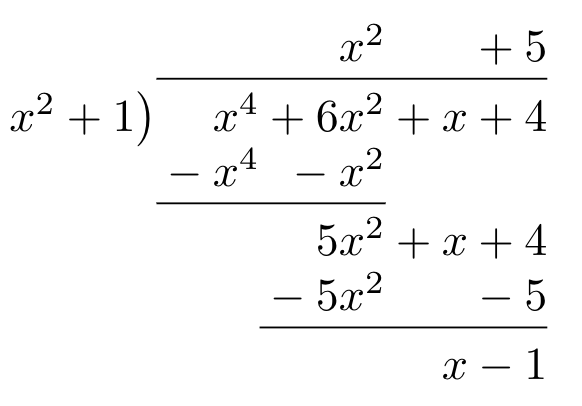So, here q(x) = x2 + 5, and r(x) = x -1.

Question 4: Given the polynomial x4 – 1. We know two of the roots are -1, 1. Find the other two roots if they exist.

Solution:

We know that the two roots are -1 and 1.

So, x -1 and x + 1 are the factors of the given polynomial. Then, (x – 1)(x +1) is also a factor of the polynomial.

(x – 1) (x + 1) = x2 – 1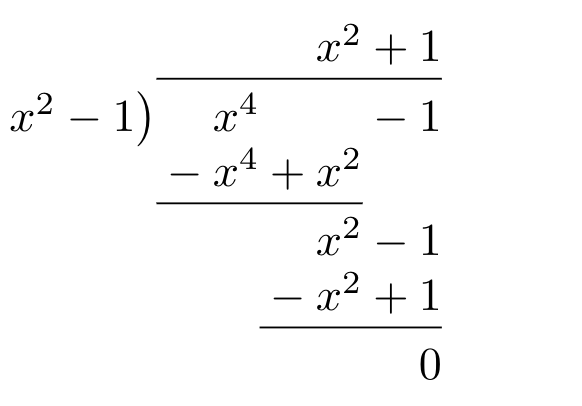We see that quotient is x2 + 1. The roots of this polynomial will be the roots of the equation.

x2 + 1 = 0

⇒ x2 = -1

This equation cannot have real roots, thus roots don’t exist for this polynomial.

Question 5: Given the polynomial p(x) = x5 + 8x3 – 6x4 + 5x2 + 10x + 8 and g(x) = x2 + 10x -5. Find q(x) and r(x).

Solution:

Using the same steps as done in previous questions,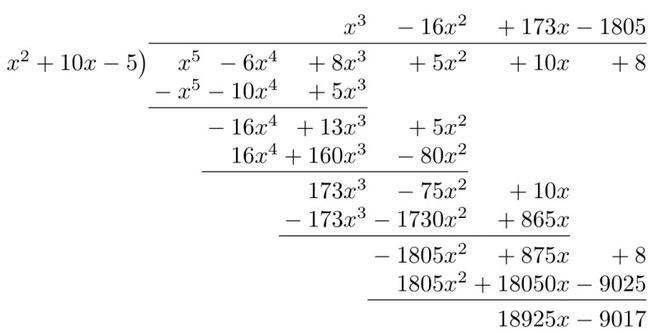q(x) = x3 – 16x2 + 173x – 1805

r(x) = 18295x – 9017

Question 6: For the same given the polynomial p(x) = x5 + 8x3 – 6x4 + 5x2 + 10x + 8 and g(x) = x + 5. Find q(x) and r(x).

Solution: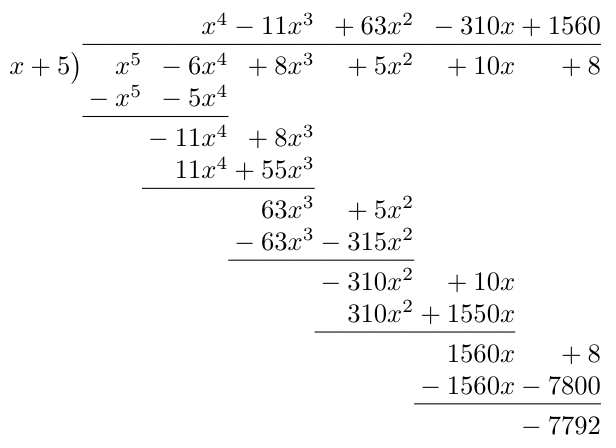Here, q(x) = x4 – 11x3 + 63x2 – 310x + 1560

r(x) = -7792

Question 7: For the same given the polynomial p(x) = x5 – 6x4 + 5x2 + 8 and g(x) = x + 2. Find q(x) and r(x).

Solution: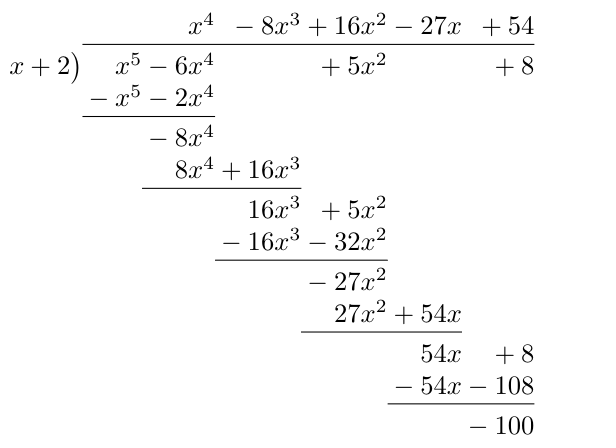q(x) = x4 – 8x3 + 16x2 -27x + 54

g(x) = -100

My Personal Notes arrow_drop_up
Related Tutorials